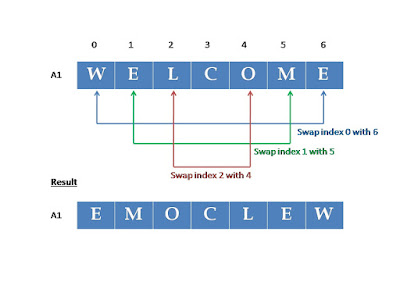Please visit, subscribe and share 10 Minutes Lectures in Computer Science

## In-place algorithm

There are devices that don’t have enough memory space. For example, mobile phones, PDA, etc. For these devices, the problem is more time is spent on I/O (input/output) operation than calculation.

In-place algorithm - an algorithm that uses a small constant amount of extra space in addition to the original input, usually overwrite the input space.

Example:

Reverse the string “WELCOME”.

Method 1:

Assume that the letters are stored in an array A1 as follows;

 W E L C O M E

To reverse the string ‘WELCOME’, we can create another array A2 and read A1 in reverse and paste each element in A2 from the beginning as follows;Non-in-place algorithm uses O(n) extra space

Drawback:
This method needs O(n) additional memory space. That is, we create another array of same size and paste the characters from the given string.

Method 2:

Assume that the letters are stored in an array A1 as follows;

 W E L C O M E

To reverse the string ‘WELCOME’, we can exchange the element stored in the first index with the last, second index with the last but one, and so on until entire string is reversed. You can observe it from the figure below;In-place algorithm - uses O(1) extra space to swap

This method implements in-place algorithm to reverse the given string. O(1) constant extra space is used to reverse the string. [This one character space is used to swap two characters from the string. Temp = A1, A1 = A1, A1 = temp]

Example in-place algorithms:

• Insertion sort
• Selection sort
Example of not-in-place (out-of-place) algorithms:

• Merge sort
******************

in-place sorting algorithm
examples for in-place algorithm
how does in-place algorithm works
how does in-place algorithm save space
how much extra space an in-place algorithm need

## Featured Content

### Multiple choice questions in Natural Language Processing Home

MCQ in Natural Language Processing, Quiz questions with answers in NLP, Top interview questions in NLP with answers Multiple Choice Que...

data recovery